• 17_NumPy数组ndarray中计算满足条件的元素的个数 连同示例代码一起说明了一种计算满足NumPy数组ndarray条件的元素数量的方法。 将描述以下内容。 全体ndarray中满足条件的元素数的计算 计算ndarray的每一行和每一列...
17_NumPy数组ndarray中计算满足条件的元素的个数
连同示例代码一起说明了一种计算满足NumPy数组ndarray条件的元素数量的方法。
将描述以下内容。

全体ndarray中满足条件的元素数的计算
计算ndarray的每一行和每一列满足条件的元素数
使用numpy.any（）（全体，行/列）检查是否有满足条件的元素
使用numpy.all（）检查所有元素是否都满足条件（全体，行/列）
多种条件

要替换满足条件的元素，请参考以下文章。

04_Numpy的函数np.where（）—满足条件的处理

全体ndarray中满足条件的元素数的计算
将ndarray与标量值进行比较将返回以布尔值（True，False）作为元素的ndarray。您可以将<，==，！=等进行比较。
import numpy as np

a = np.arange(12).reshape((3, 4))
print(a)
# [[ 0  1  2  3]
#  [ 4  5  6  7]
#  [ 8  9 10 11]]

print(a < 4)
# [[ True  True  True  True]
#  [False False False False]
#  [False False False False]]

print(a % 2 == 1)
# [[False  True False  True]
#  [False  True False  True]
#  [False  True False  True]]

使用np.count_nonzero（）获得True的数量，即满足条件的元素的数量
print(np.count_nonzero(a < 4))
# 4

print(np.count_nonzero(a % 2 == 1))
# 6

Trueは1, Falseは0として扱われるのでnp.sum()を使うことも可能。ただし、np.count_nonzero()のほうが高速。
print(np.sum(a < 4))
# 4

print(np.sum(a % 2 == 1))
# 6

计算ndarray的每一行和每一列满足条件的元素数
通过指定参数轴，为每个轴（每个维度）处理多维数组的Np.count_nonzero（）。
在二维数组的情况下，将在参数axis= 0的列中进行处理，在axis= 1的行中进行处理。通过使用它，可以计算满足每一行和每一列条件的元素的数量。
print(np.count_nonzero(a < 4, axis=0))
# [1 1 1 1]

print(np.count_nonzero(a < 4, axis=1))
# [4 0 0]

print(np.count_nonzero(a % 2 == 1, axis=0))
# [0 3 0 3]

print(np.count_nonzero(a % 2 == 1, axis=1))
# [2 2 2]

自版本1.12.0起，可以使用np.count_nonzero（）指定参数轴。由于np.sum（）自1.7.0版以来已实现参数轴，因此可以将np.sum（）用于较早的版本。
使用numpy.any（）（全体，行/列）检查是否有满足条件的元素
np.any（）是一个函数，如果在作为第一个参数传递的ndarray中至少有一个True元素，则返回True，否则返回False。
print(np.any(a < 4))
# True

print(np.any(a > 100))
# False

与np.count_nonzero（）类似，如果指定了参数轴，则np.any（）将处理每一行和每一列。
print(np.any(a < 4, axis=0))
# [ True  True  True  True]

print(np.any(a < 4, axis=1))
# [ True False False]

使用numpy.all（）检查所有元素是否都满足条件（全体，行/列）
np.all（）是一个函数，如果在第一个参数中传递的ndarray的所有元素均为True，则返回True，否则返回False。
print(np.all(a < 4))
# False

print(np.all(a < 100))
# True

与np.count_nonzero（）相似，当指定参数轴时，将对每一行和每一列处理np.all（）。
print(np.all(a < 4, axis=0))
# [False False False False]

print(np.all(a < 4, axis=1))
# [ True False False]


多种条件
如果要组合多个条件，请将每个条件表达式括在（）中，并将其与＆或|连接。
print((a < 4) | (a % 2 == 1))
# [[ True  True  True  True]
#  [False  True False  True]
#  [False  True False  True]]

print(np.count_nonzero((a < 4) | (a % 2 == 1)))
# 8

print(np.count_nonzero((a < 4) | (a % 2 == 1), axis=0))
# [1 3 1 3]

print(np.count_nonzero((a < 4) | (a % 2 == 1), axis=1))
# [4 2 2]

请注意，如果省略括号（）或使用and和or，将发生错误。


展开全文python numpy
• excel中如何计算个数，使用这个方法，给出汉字描述，自动生成Excel统计公式。支持Excel中分类统计数量 ... ... 选择下面的其中一个： ... 查询满足条件的行的数量（个数） ... 查询满足条件行及列的（满足条件...
excel中如何计算个数，使用这个方法，给出汉字描述，自动生成Excel统计公式。支持Excel中分类统计数量

地址：http://www.excelfb.com/

点击：Excel自动写公式工具（根据汉字描述写公式,支持合并单元格公式）

选择下面的其中一个：

查询满足条件的行的数量（个数）

查询满足条件的单元格的数量（个数）

查询满足条件行及列的（满足条件的）单元格的数量（个数）

操作如下图：

一、  求满足条件的行的数量（个数）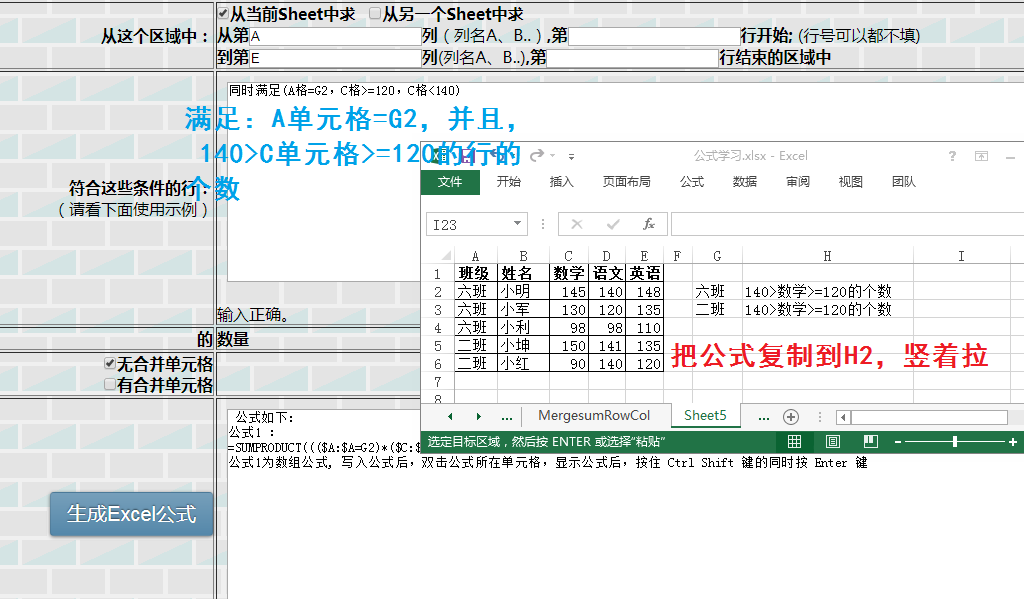二、求满足条件的单元格的数量（个数）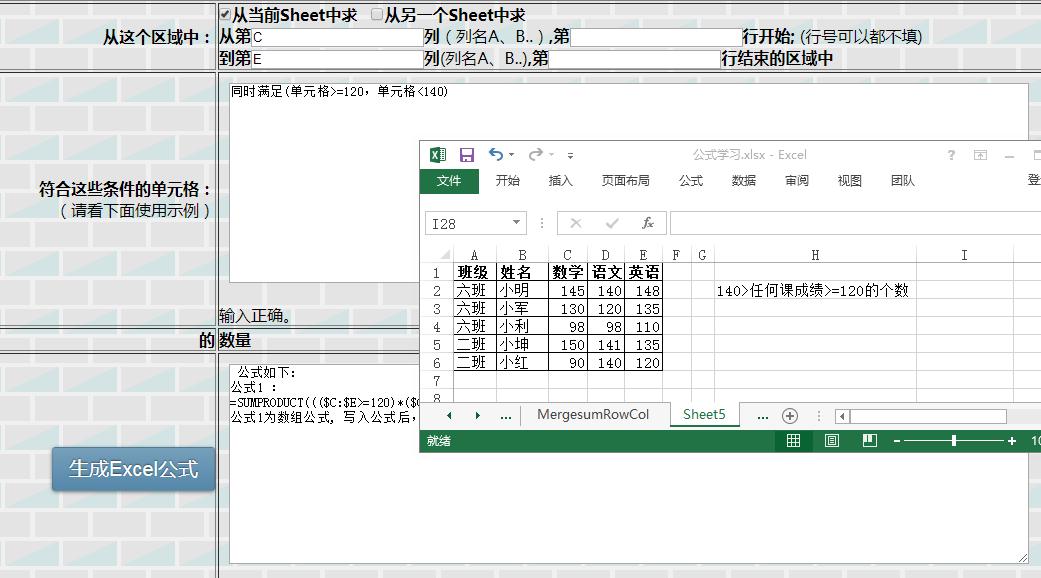三、求满足条件行及列的（满足条件的）单元格的数量（个数）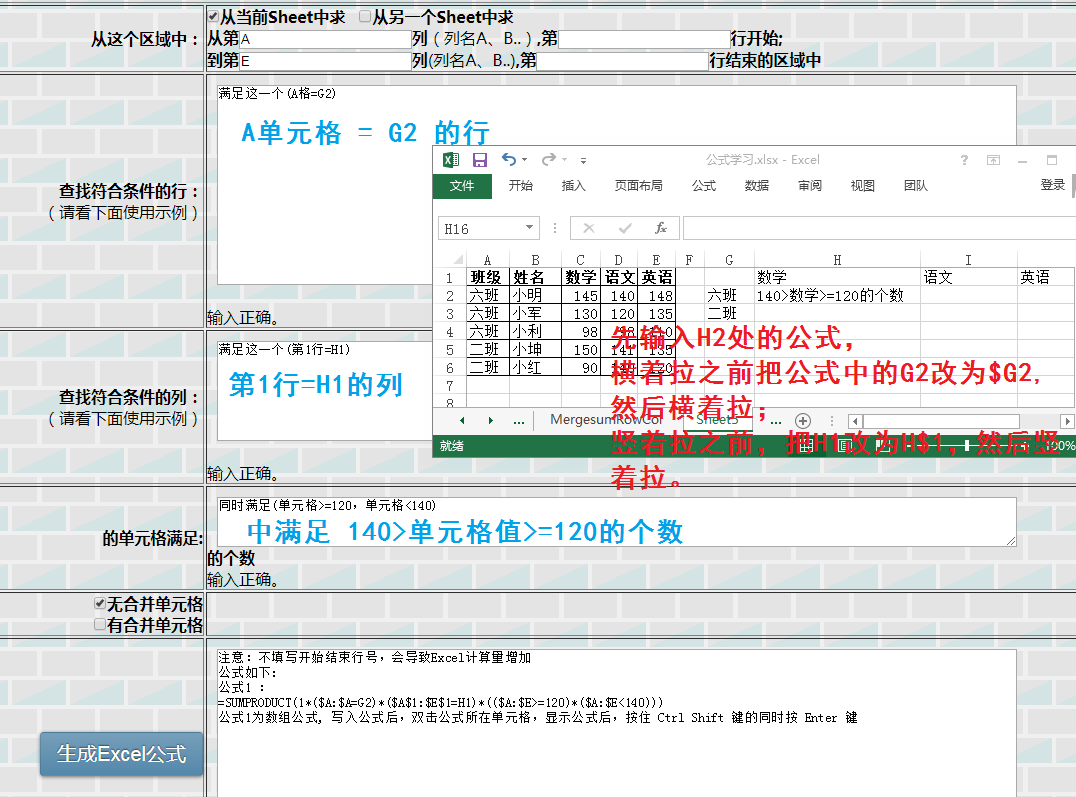展开全文• 要求：统计list weights['value']中满足条件的数的个数： &gt;=0、&lt;0的个数分别是多少 解决方法： np.sum(list(map(lambda x: x &gt;= 0, weights['value'])))
要求：统计list weights['value']中满足条件的数的个数：

>=0、<0的个数分别是多少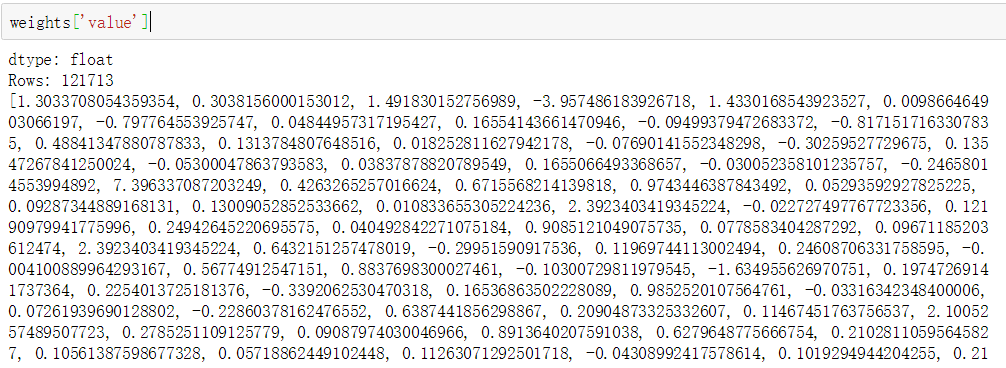解决方法：

np.sum(list(map(lambda x: x >= 0, weights['value'])))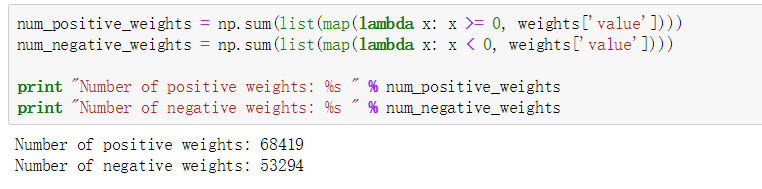展开全文• 我们要求找出具有下列性质数的个数(包含输入的自然数 n)。先输入一个自然数n(n&lt;=1000),然后对此自然数按照如下方法进行处理:1.不作任何处理;2.在它的左边加上一个自然数,但该自然数不能超过原数最高位数字的...
我们要求找出具有下列性质数的个数(包含输入的自然数 n)。先输入一个自然数n(n<=1000),然后对此自然数按照如下方法进行处理:1.不作任何处理;2.在它的左边加上一个自然数,但该自然数不能超过原数最高位数字的一半;3.加上数后,继续按此规则进行处理,直到不能再加自然数为止。

【输入】  第一行一个数t，表示有t组数据，之后每组数据占一行，每行一个数 n 。

【输出】  每组数据占一行，一个数，表示满足条件的数的个数。

【输入范例】1 6

【输出范例】6

程序代码：

#include<stdio.h>
int main()
{
int n,t,a; //n表示输入的数，t表示有t组数据，a表示满足条件的数的个数
scanf("%d",&t);      //输入t
while(t--) //进入循环，可以输入t组数据
{
scanf("%d",&n);             //输入n
a = 1;       //因为满足条件的数中包含该数本身，所以a赋初值为1
while(n-10 >= 0)
{   /*因为要对根据该数的最高位进行判断，所以，如果该数等于10即为两位数或高位数，则进入循环，取出该数的最高位*/
n = n/10;
}
for(int i=1;i <= n/2;i++)   //第一个for循环计算对该数进行第一次处理后满足条件的数的个数
{
a = a+1;               //找到一个对a加1
for(int j=1;j<=i/2;j++)     //第二个for循环计算对第一次处理后满足条件的数进行第二次处理后满足条件的数的个数
{
a=a+1;            //找到一个对a加1
for(int k=1;k <= j/2;k++)  //第三个for循环计算对第二次处理后满足条件的数进行第三次处理后满足条件的数的个数
{
a=a+1;        //找到一个对a加1
}
}
}
printf("%d\n",a); //输出
}
return 0;
}


展开全文• ## 计算二叉树的个数

千次阅读 2018-08-04 09:56:32
说明 给定二叉树的节点个数来计算能够构成多少种不同的二叉树。 操作 ...即满足这样的条件，从根节点下来，能够成左边的情况的个数乘以右边的个数的情况想乘，就得到此时这种情况的个数。例如...
• 设计一个时间复杂度为O(N)的算法，找出满足下面两个条件的数对的个数: 1) 相邻两个数的和也在这在这个数组之中; 2) 这两个数本身也相邻。 答: 这个数组中的数有几个特征:(1) 每个都不相同；(2) 取值...算法 c
• 35_Pandas计算满足特定条件的元素的数量 将说明在pandas.DataFrame和pandas.Series中，如何按行/列以及整体来计算满足特定条件的元素数。 请参阅以下文章，了解如何提取符合条件的行。 09_Pandas从多个条件（AND，...python 机器学习 pandas
• ## 计算1的个数

千次阅读 2010-11-05 20:03:00
给定一个十进制正整数N，写下从1开始，到N的所有整数，然后数一下其中出现的所有“1”的个数。 例如： N= 2，写下1，2。这样只出现了1个“1”。... 在32位整数范围内，满足条件“f算法 c
• 试题描述 给定若干个四位数，求出其中满足以下条件的数的个数： 个位数上的数字减去千位数上的数字，再减去百位数...输出为一行，包含一个整数，表示满足条件的四位数的个数。 样例输入 5 1234 1349 6119 2123 5017C语言入门
• 勾股定理，西方称为毕达哥拉斯定理，它所对应的三角形现在称为：直角三角形。...要求输出一个整数，表示满足条件的直角三角形个数。 例如，输入： 5 程序应该输出： 1 再例如，输入： 100 程序应该输出：
• 输出区间[M,N](10≤M≤N≤1000)之间所有各位数值之和为k的数，并统计输出满足条件的数的个数。 输入格式: 输入在第一行中给出正整数k，第二行中给出2个正整数M和N(10≤M≤N≤1000)，中间用空格间隔。 输出格式: 在...算法 c++
• 请编制一个函数jsVal()，其功能是：依次从数组a中取出一个四位数，如果该四位数连续大于该四位数以后的5个数，且该数是奇数，则把这个四位数按从小到大的顺序存入数组b中，并计算满足上述条件的四位数的个数cnt。...fp file
• 描述： 给定若干个四位数，求出其中满足以下条件的数的个数：  个位数上的数字减去千位数上的数字，再减去百位数上的数字...输出为一行，包含一个整数，表示满足条件的四位数的个数。 样例输入： 5 1234 13...
• 如何计算数组中某项的个数<script type="text/javascript"> //统计一个数组中有多少个指定的数据项 var arr1= [12, 5, 8, 130, 44,5,51,5]; //方法一： function count(arr,num){ var total = 0; for (var i = ...数组 filter find forEach
• select count(*) as 总数, sum(1) as 所有条数，sum(a=2) as 符合条件的条数，sum(a=1) as 符合条件的条数 from 表 group by 字段
• 大马驮3担，中马驮2担，两匹小马驮1担，输出所有满足条件大、中、小马的个数。 输入 无 输出 输出有多行，每行表示一组符合条件的数据；每行输出大马，中马，小马的个数，中间用空格隔开。 多行输出按照大马的数目由...
• 要求输出一个整数，表示满足条件的直角三角形个数。例如，输入：5程序应该输出：1再例如，输入：100程序应该输出：2再例如，输入：3程序应该输出：0资源约定：峰值内存消耗（含虚拟机） &lt; 25...Java
•函数 Angular4 JavaScript
• 求出满足下列条件的4位数： 1、该数是完全平方数 2、且第一位和第三位数字之和为10 3、第二位与第四位数字之积为12 import math for num in range(1000,10000): #生成4位数 if len(str(math.sqrt(num))) == 4...Python题目
• 习题10-1 判断满足条件的三位数 (15分) 本题要求实现一个函数，统计给定区间内的三位数中有两位数字相同的完全平方数（如...函数search返回[101, n]区间内所有满足条件的数的个数。 裁判测试程序样例： #in
• 设计一个算法，找出数列中符合条件的数对的个数满足数对中两数的和等于N+1。 方法一：排序＋二分查找。先对数组进行排序，然后遍历该有序数组，同时使用二分查找方法，查找对应的值是否存在（例如，有序数组中某...hash法
• 输出为一行，包含一个整数，表示满足条件的四位数的个数。 【输入样例】 5 1234 1349 6119 2123 5017 【输出样例】 3 ` #include #include using namespace std; int main() {char a,b,c,d; int n,y...c++ for循环
• 引言 总体策略 不要62 Bomb B-number Balanced Number引言 在算法竞赛中，有一类求出给定区间中符合要求的数的个数问题，这类问题往往区间范围较大，...求解[l,r][l, r]满足条件的数个数可以通过[0,r]−[0,l−1][0,算法竞赛 数位dpfp file
• iamlaosong文 要求从货运明细中统计各省某日发货点数量，公式如下： =SUMPRODUCT((Sheet1!$L$2:$L$1747=B6) * (Sheet1!$M$2:$M1747=$A$1) / COUNTIF(Sheet1!$U$2:$U$1747,Sheet1!$U$2:$U\$1747)) ...
• ## 计算n阶乘中尾部零的个数

万次阅读 多人点赞 2016-04-16 15:17:08
写在前面本来觉得问题挺容易的，...这里只是记录一下自己最近两天对此问题的一些想法，目前只能说是解决了问题，并且满足题目要求。据说问题来自《编程之美》，以后刷书本的时候看到原题，如果需要补充的话，再来更新。算法 时间复杂度
• 本题要求实现一个函数，统计给定区间内的三位数中有两位数字相同的完全平方数（如144、...函数search返回[101, n]区间内所有满足条件的数的个数。 裁判测试程序样例： #include <stdio.h> #include <math.h...
• 6-3 判断满足条件的三位数 (15 分) 本题要求实现一个函数，统计给定区间内的三位数中有两位数字相同的完全平方...函数search返回[101, n]区间内所有满足条件的数的个数。 裁判测试程序样例： #include <stdio.h&g...C语言...